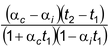# Thermal Expansion

## Heat And Thermodynamics of Class 11

Experiments show that most of bodies increase their volume upon heating. The extent of expansion of various bodies is characterized by the temperature coefficient of expansion, or simply the coefficient of expansion. While considering solid which retain their shape during temperature variations, the distinction is made between (a) a change in their linear dimensions (viz. the dimensions in a certain direction), i.e. linear expansion, and (b) a change in the volume of a body, i.e. cubic expansion.

The coefficient of linear expansion is the quantity α equal to the fraction of the initial length by which a body taken at 0oC has elongated as a result of heating it by 1oC (or by 1 K):

α = (lt – lo)/lot

where lo is the initial length at 0oC and lt is the length at a temperature t. From this expansion, we can find

lt = lo(1 + αt)

The dimensions of α are K-1 (or oC-1).

The coefficient of cubic expansion is the quantity γ equal to the fraction of the initial volume by which the volume of a body taken at 0oC has increased upon heating it by 1oC (or by 1 K):

γ = (Vt – Vo)/Vot,

where Vo is the volume of a body at 0oC and Vt is its volume at a temperature t. From this equation, we obtain

Vt = Vo(1 + γt)

The quantity γ has also the dimensions of K-1 (or oC-1).

The coefficient of cubic expansion is about three times larger than the coefficient of linear expansion:

γ = 3α

The coefficients of γ of cubic expansion for liquids are somewhat higher than for solid bodies, ranging between 10-3 and 10-4 K-1.

What obeys the general laws of thermal expansion only at a temperature above 4 oC. From 0 oC to
4 oC, water contracts rather than expands. At 4 oC, water occupies the smallest volume, i.e. it has the highest density. At the bottom of deep lakes, there is denser water in winter, which remains the temperature of 4 oC even after the upper layer has been frozen.

Example 5.1

The lengths l1i = 100 m of iron wire and l1c = 100 m of copper wire are marked off at t1 = 20 oC. What is the difference in lengths of the wires at t2 = 60 oC? The coefficients of linear expansion for iron and copper are α1 = 1.2 × 10-5 K-1 and αc = 1.7 × 10-5 K-1.

Solution

l1i = lo(1 + αit1) and l2i = loi(1 + αit2)

The elongation of the iron wires is

l2i – l1i = loi(t2 – t1).

Substituting loi = l1i/(1 + fit1), we find the elongation of the iron and copper wires

l2i – l1i = l1iαi(t2 – t1)/(1 + αit1)(1)

l2c – l1c = l1cαc(t2 – t1)/(1 + αct1)(2)

Subtracting (1) from (2) and considering that l1i = l1c = l1, we obtain

l2c – l2i = l1= 19.9 mm

For low values of temperature t, when αt < 1, it is not necessary to reduce l1 and l2 to lo1 and lo2 at t = 0 oC. To a sufficiently high degree of accuracy, we an assume that Δl = lαΔt. Under this assumption, the problem can be solved in a simpler way:

Δli = l1iαi(t2 – t1), Δlc = l1cαc(t2 – t1)

Consequently, since l1i = l1c = l1, we have

Δl = Δlc - Δli = l1(t2 – t1)(αc - αi) = 20 mm

It can been seen that the deviation from a more exact value of 19.9 mm amounts to 0.1 mm, i.e. the relative error Δ = 0.1/19.9 = 0.5%.

Example 5.2

A solid body floats in a liquid at a temperature t = 0o C and is completely submerged in it at 50o C. What fraction δ of volume of the body is submerged in the liquid at 0o C if
γs = 0.3 × 10-5 K-2 and of the liquid, γ1 = 8.0 × 10-5 K-1?

Solution

In both the cases the weight of the body will be balanced by the force of buoyancy on it.

At to = 0 oC, the buoyancy is Fb = δVoρog (1)

where Vo is the volume of the body and ρo is the density of the liquid at to = 0oC. At t = 50 oC, the volume of the body becomes V = Vo(1 + γst) and the density of the liquid is ρ1 = ρo/(1 + γ1t). The buoyancy in this case is

Fb = Voρog(1 + γst)/(1 + γ1t) (2)

Equating the right-hand sides of equation (1) and (2), we get

δ = (1 + γst)/(1 + γ1t) = 96%